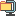검색어 입력폼

# 수치해석

등록일 2000.09.21 | 최종수정일 2014.05.09압축파일 (arj) | 18페이지 | 가격 10,000원

## 소개글

수치해석에 대한 연습문제 풀이입니다. 다소 까다로운 과목이라 소스가 필요할 실 거예요.

## 목차

1) problem definition
1. description
2. problems

2) given parameters

3) theoretical analysis
1. equation derivation
2. derivation of linear algebraic equations set
3. evaluation of coefficients Aij and forcing terms Qi
4. pressure calculation
5. mass flow rate calculation
6. Reynolds number checking

4) program schema
1. variables
2. analysis steps

5) numerical results
1. linear algebraic equations set
2. pressure (N/cm2 gauge)
3. flow rate (gm/sec) : vij flow rate from node i to node j
4. Reynolds number : Re ij Reynolds number from node i to node j

6) discussion
1. distribution analysis

7) program list

8) diskette file list

## 본문내용

1. description
There is a lubrication system of a machine. It has 1 inlet and 12 outlets. The pipes are one of 4 types - A, B, C, and F.
The flow is laminar in the tubes between each node, and the relation between pressure drop and flow in each line is given by where V is the (mean) velocity in the tube.

2. problems
(1) derive Eq.(1)

(2) use a mass balance at each point and Eq.(1) to derive a set of linear algebraic equations for the pressure at the nodes. Neglect turning and expansion losses. Do the analysis in symbolic terms i.e. in terms of Pi, Pj, Dij, Vij, etc, where ij is the line connecting nodes i and j. Work your equations for the ith node into the form

(3) evaluate the coefficients Aij and the forcing terms Qi. Then calculate the pressure at each node using the subroutines in the text or equivalent ones.
(4) have your program calculate the mass flow rate (gm/sec) in each lines. draw the direction and magnitude of these flows on the sketch, and turn it in with your analysis.
(5) The Reynolds number is a non dimensional group defined by . for flow to be laminar we must have Re<2000. check whether this is the case in each line (have the computer do this for you).

없음